# RD Sharma Solutions for Class 10 Maths Chapter 8 Quadratic Equations Exercise 8.8

Applications of quadratic equations are found in many areas of study and applications. One of these is solving problems on time and distance. In order to clear any conceptual doubts regarding these refer to the RD Sharma Solutions Class 10. More detailed solutions of the RD Sharma Solutions for Class 10 Maths Chapter 8 Quadratic Equations Exercise 8.8 given in the PDF below.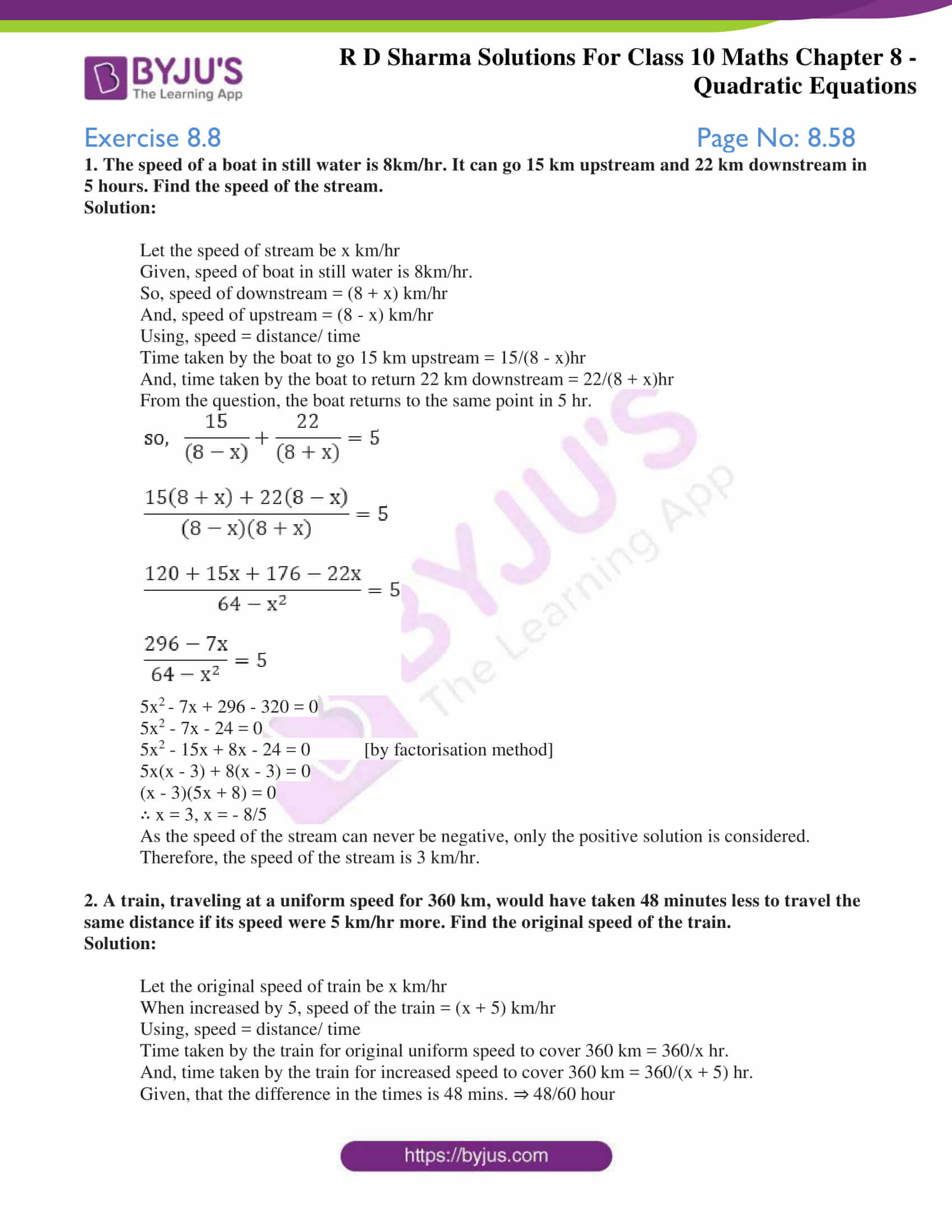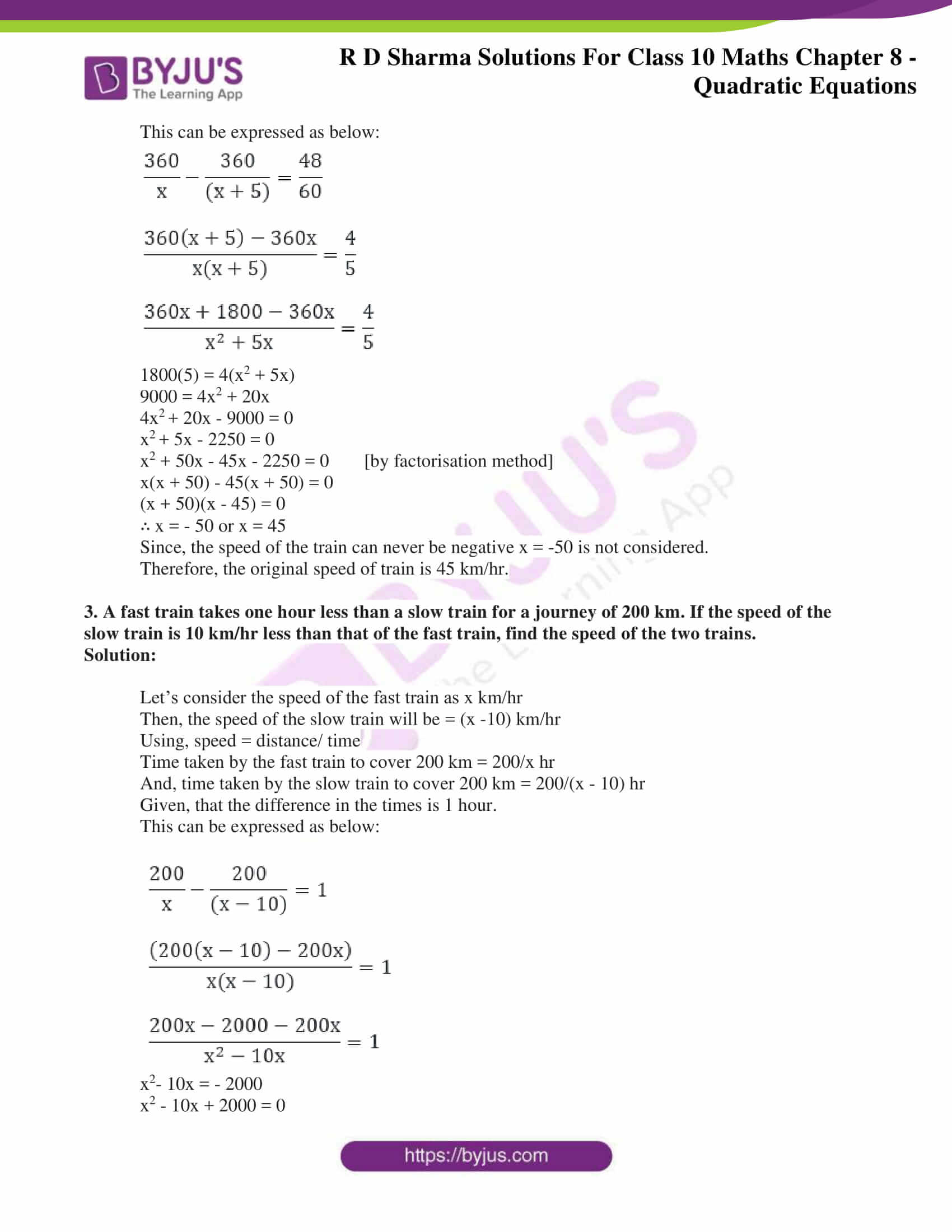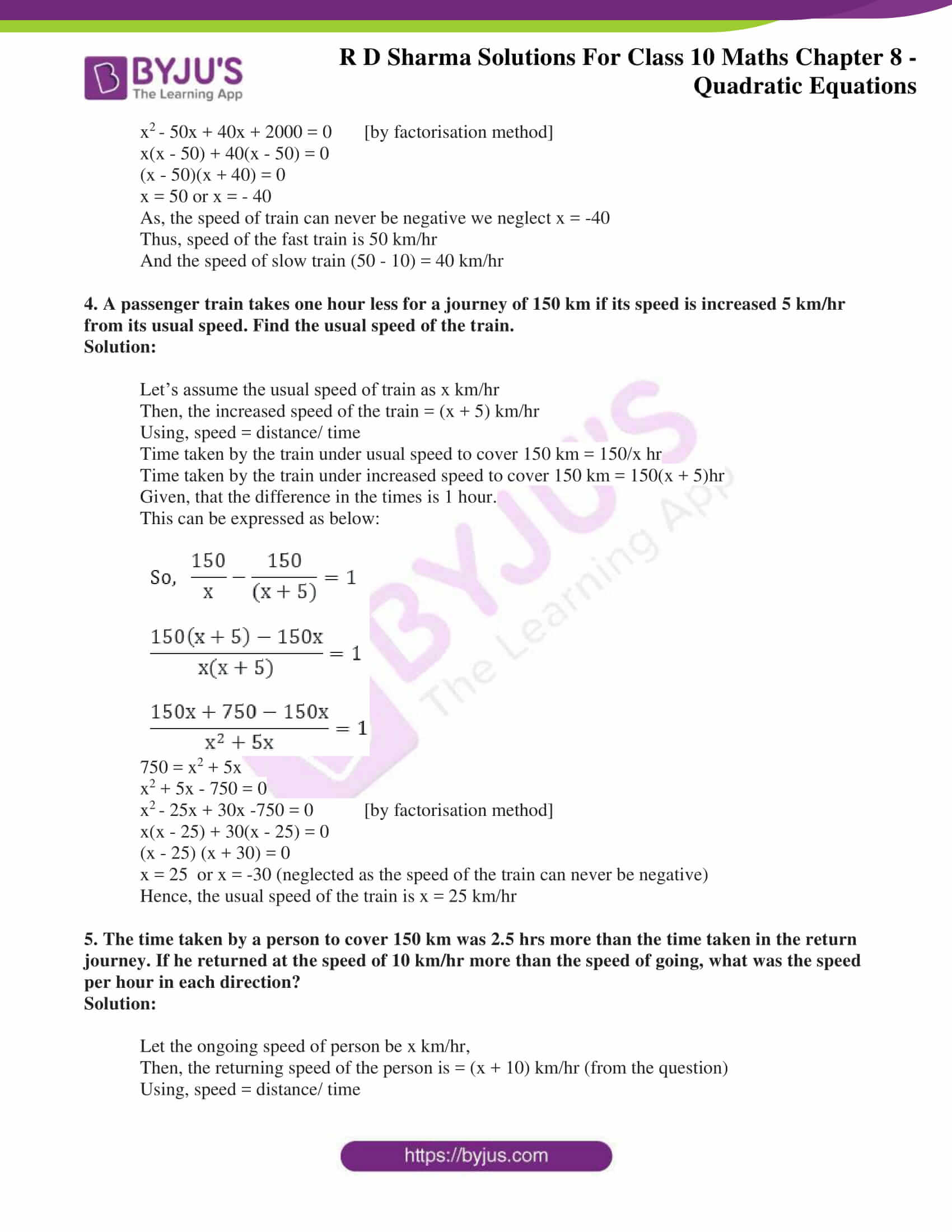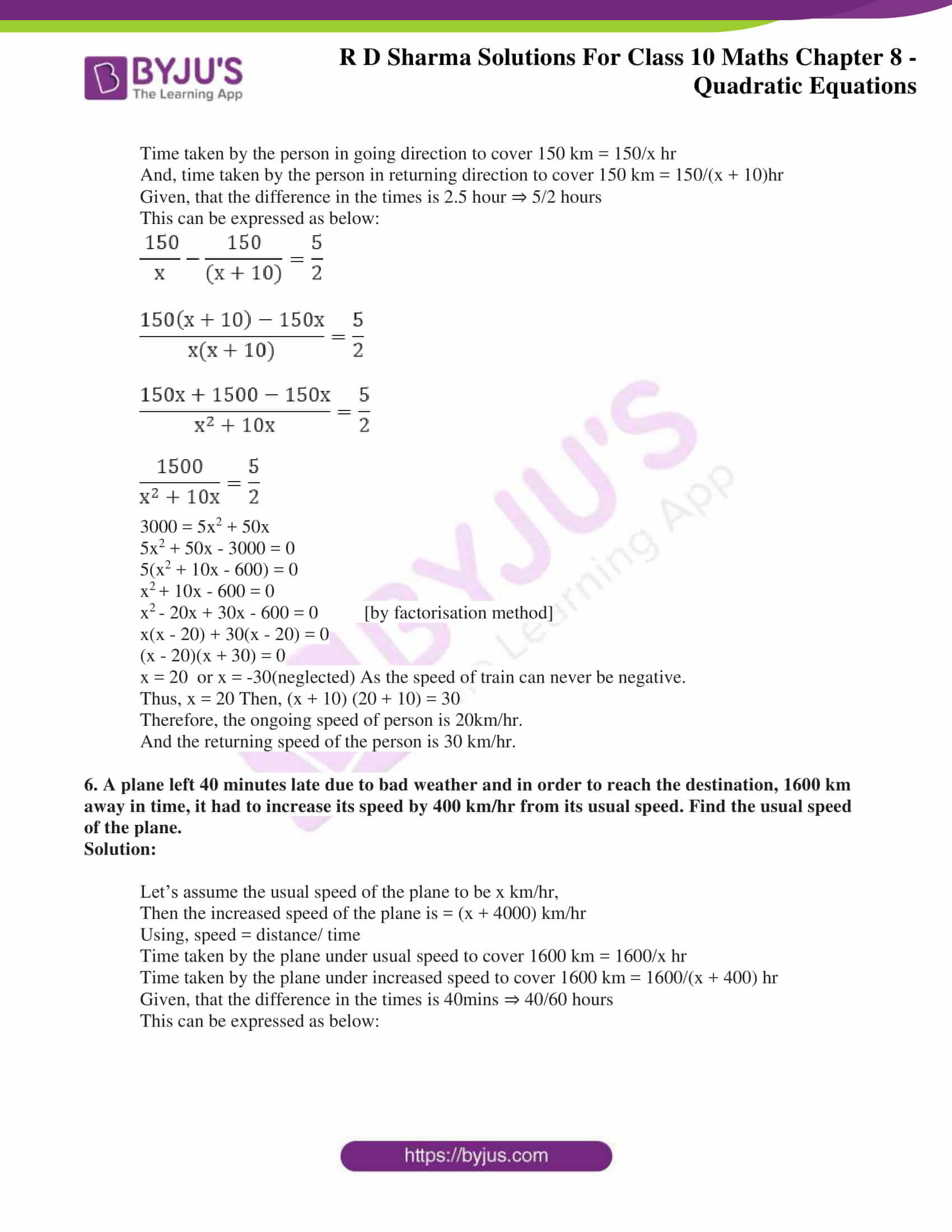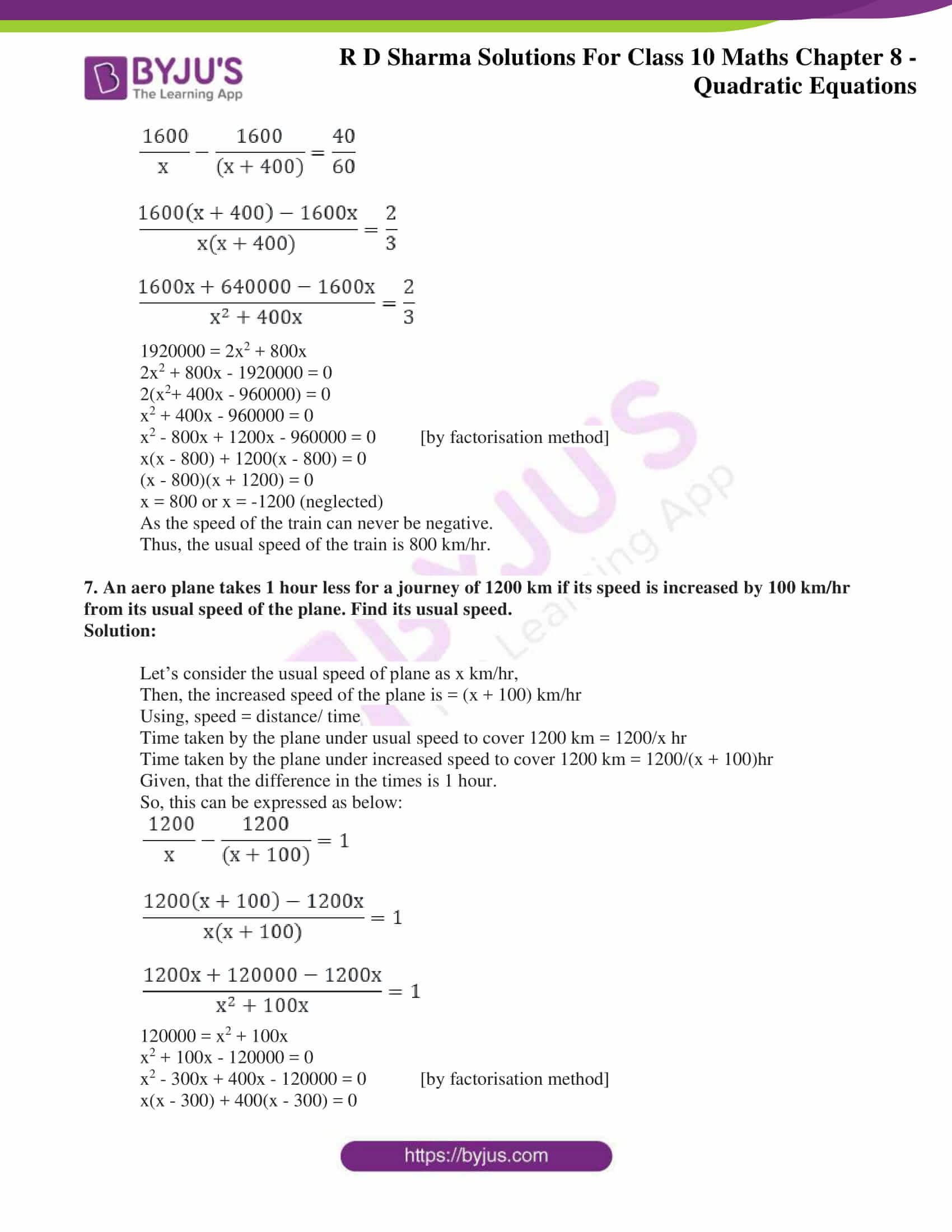### Access RD Sharma Solutions for Class 10 Maths Chapter 8 Quadratic Equations Exercise 8.8

1. The speed of a boat in still water is 8km/hr. It can go 15 km upstream and 22 km downstream in 5 hours. Find the speed of the stream.

Solution:

Let the speed of stream be x km/hr

Given, speed of boat in still water is 8km/hr.

So, speed of downstream = (8 + x) km/hr

And, speed of upstream = (8 – x) km/hr

Using, speed = distance/ time

Time taken by the boat to go 15 km upstream = 15/(8 – x)hr

And, time taken by the boat to return 22 km downstream = 22/(8 + x)hr

From the question, the boat returns to the same point in 5 hr.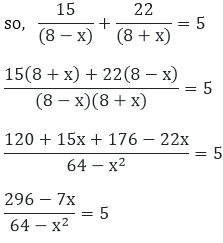5x– 7x + 296 – 320 = 0

5x2 – 7x – 24 = 0

5x2 – 15x + 8x – 24 = 0 [by factorisation method]

5x(x – 3) + 8(x – 3) = 0

(x – 3)(5x + 8) = 0

∴ x = 3, x = – 8/5

As the speed of the stream can never be negative, only the positive solution is considered.

Therefore, the speed of the stream is 3 km/hr.

2. A train, traveling at a uniform speed for 360 km, would have taken 48 minutes less to travel the same distance if its speed were 5 km/hr more. Find the original speed of the train.

Solution:

Let the original speed of train be x km/hr

When increased by 5, speed of the train = (x + 5) km/hr

Using, speed = distance/ time

Time taken by the train for original uniform speed to cover 360 km = 360/x hr.

And, time taken by the train for increased speed to cover 360 km = 360/(x + 5) hr.

Given, that the difference in the times is 48 mins. ⇒ 48/60 hour

This can be expressed as below: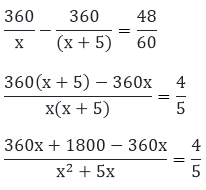1800(5) = 4(x2 + 5x)

9000 = 4x2 + 20x

4x+ 20x – 9000 = 0

x+ 5x – 2250 = 0

x2 + 50x – 45x – 2250 = 0 [by factorisation method]

x(x + 50) – 45(x + 50) = 0

(x + 50)(x – 45) = 0

∴ x = – 50 or x = 45

Since, the speed of the train can never be negative x = -50 is not considered.

Therefore, the original speed of train is 45 km/hr.

3. A fast train takes one hour less than a slow train for a journey of 200 km. If the speed of the slow train is 10 km/hr less than that of the fast train, find the speed of the two trains.

Solution:

Let’s consider the speed of the fast train as x km/hr

Then, the speed of the slow train will be = (x -10) km/hr

Using, speed = distance/ time

Time taken by the fast train to cover 200 km = 200/x hr

And, time taken by the slow train to cover 200 km = 200/(x – 10) hr

Given, that the difference in the times is 1 hour.

This can be expressed as below: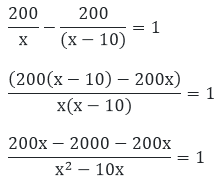x2– 10x = – 2000

x2 – 10x + 2000 = 0

x– 50x + 40x + 2000 = 0 [by factorisation method]

x(x – 50) + 40(x – 50) = 0

(x – 50)(x + 40) = 0

x = 50 or x = – 40

As, the speed of train can never be negative we neglect x = -40

Thus, speed of the fast train is 50 km/hr

And the speed of slow train (50 – 10) = 40 km/hr

4. A passenger train takes one hour less for a journey of 150 km if its speed is increased 5 km/hr from its usual speed. Find the usual speed of the train.

Solution:

Let’s assume the usual speed of train as x km/hr

Then, the increased speed of the train = (x + 5) km/hr

Using, speed = distance/ time

Time taken by the train under usual speed to cover 150 km = 150/x hr

Time taken by the train under increased speed to cover 150 km = 150(x + 5)hr

Given, that the difference in the times is 1 hour.

This can be expressed as below: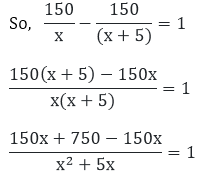750 = x2 + 5x

x2 + 5x – 750 = 0

x– 25x + 30x -750 = 0 [by factorisation method]

x(x – 25) + 30(x – 25) = 0

(x – 25) (x + 30) = 0

x = 25  or x = -30 (neglected as the speed of the train can never be negative)

Hence, the usual speed of the train is x = 25 km/hr

5. The time taken by a person to cover 150 km was 2.5 hrs more than the time taken in the return journey. If he returned at the speed of 10 km/hr more than the speed of going, what was the speed per hour in each direction?

Solution:

Let the ongoing speed of person be x km/hr,

Then, the returning speed of the person is = (x + 10) km/hr (from the question)

Using, speed = distance/ time

Time taken by the person in going direction to cover 150 km = 150/x hr

And, time taken by the person in returning direction to cover 150 km = 150/(x + 10)hr

Given, that the difference in the times is 2.5 hour ⇒ 5/2 hours

This can be expressed as below: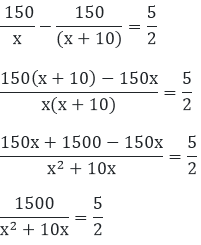3000 = 5x2 + 50x

5x2 + 50x – 3000 = 0

5(x2 + 10x – 600) = 0

x+ 10x – 600 = 0

x– 20x + 30x – 600 = 0 [by factorisation method]

x(x – 20) + 30(x – 20) = 0

(x – 20)(x + 30) = 0

x = 20  or x = -30(neglected) As the speed of train can never be negative.

Thus, x = 20 Then, (x + 10) (20 + 10) = 30

Therefore, the ongoing speed of person is 20km/hr.

And the returning speed of the person is 30 km/hr.

6. A plane left 40 minutes late due to bad weather and in order to reach the destination, 1600 km away in time, it had to increase its speed by 400 km/hr from its usual speed. Find the usual speed of the plane.

Solution:

Let’s assume the usual speed of the plane to be x km/hr,

Then the increased speed of the plane is = (x + 4000) km/hr

Using, speed = distance/ time

Time taken by the plane under usual speed to cover 1600 km = 1600/x hr

Time taken by the plane under increased speed to cover 1600 km = 1600/(x + 400) hr

Given, that the difference in the times is 40mins ⇒ 40/60 hours

This can be expressed as below: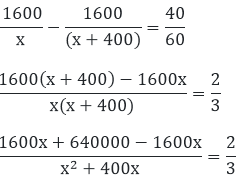1920000 = 2x2 + 800x

2x2 + 800x – 1920000 = 0

2(x2+ 400x – 960000) = 0

x2 + 400x – 960000 = 0

x2 – 800x + 1200x – 960000 = 0 [by factorisation method]

x(x – 800) + 1200(x – 800) = 0

(x – 800)(x + 1200) = 0

x = 800 or x = -1200 (neglected)

As the speed of the train can never be negative.

Thus, the usual speed of the train is 800 km/hr.

7. An aero plane takes 1 hour less for a journey of 1200 km if its speed is increased by 100 km/hr from its usual speed of the plane. Find its usual speed.

Solution:

Let’s consider the usual speed of plane as x km/hr,

Then, the increased speed of the plane is = (x + 100) km/hr

Using, speed = distance/ time

Time taken by the plane under usual speed to cover 1200 km = 1200/x hr

Time taken by the plane under increased speed to cover 1200 km = 1200/(x + 100)hr

Given, that the difference in the times is 1 hour.

So, this can be expressed as below: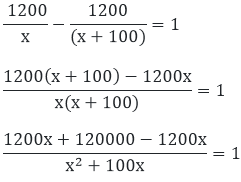120000 = x2 + 100x

x2 + 100x – 120000 = 0

x2 – 300x + 400x – 120000 = 0 [by factorisation method]

x(x – 300) + 400(x – 300) = 0

x = 300 or x = – 400 neglected as the speed of the aero plane can never be negative.

Therefore, the usual speed of train is 300 km/hr.